# Abel-Goncharov problem

(Redirected from Abel–Goncharov problem)

Goncharov problem

A problem in the theory of functions of a complex variable, consisting of finding the set of all functionsfrom some class satisfying the relations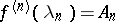(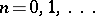). Hereandare sequences of complex numbers admissible for the given class. The problem was posed by V.L. Goncharov .

A functionis put in correspondence with the series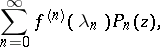(*)

the Abel–Goncharov interpolation series, where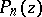is the Goncharov polynomial defined by the equalities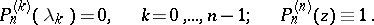N.H. Abel gave a formal treatment of the case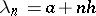,whereand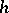are real numbers(cf. ). Here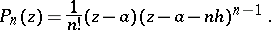The series (*) is used to study the zeros of the successive derivatives of regular functions. The set of functions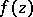representable by a series (*) is called the convergence class of the Abel–Goncharov problem.

In the case when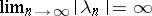, the Abel–Goncharov convergence class has been expressed in terms of bounds on the order and the type of entire functions, depending on the growth of the quantity.

If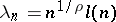, where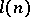is a slowly increasing function,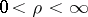, the Abel–Goncharov convergence class has, in a sense, been exactly determined . Other Abel–Goncharov convergence classes for entire functions of finite and infinite order have been identified in terms of various constraints on the indicators of the respective classes of functions. The Abel–Goncharov problem has also been studied for entire functions of several variables. Exact estimates of Goncharov polynomials were obtained for some classes of interpolation nodes.

Let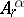be the class of functionsof the form, and let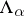be the class of all possible sequences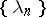such that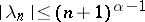,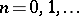. By definition, the bound of convergence for the classis the supremum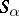of the values offor which every function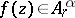can be represented by a series (*). The infimum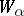of the-values for which there exist a functionand a sequence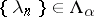such that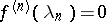,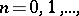, is called the bound of uniqueness. The quantities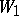and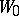are known, respectively, as the Whittaker constant and the Goncharov constant. It has been shown that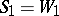(cf. ); the more general statementshave also been proved , .

Thus, if, the Abel–Goncharov problem is reduced to finding the constant. Its precise numerical value is not known, but one has obtained the bounds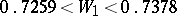.

Relative to the Abel–Goncharov problem for the classof functions which are regular in the regionand such that, the following has been shown: For any set of numbers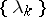,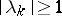, which satisfy the condition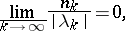where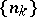is an increasing sequence of natural numbers, the equations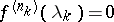,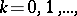imply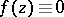. Moreover, for any numberthere exist a sequenceand a function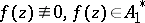, for which,.

The Abel–Goncharov problem includes the so-called two-point problem posed by J.M. Whittaker . Let the sequences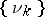and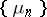be such that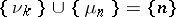and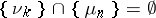. The problem is to determine conditions under which there exists a function, regular on the segment, satisfying the conditions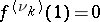,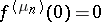. This problem was solved for various subclasses of the class of functions regular in the disc(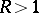). The resulting conditions, which are in a sense precise, are expressed in terms of various bounds imposed on the coefficients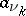of the expansions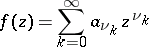depending on. This problem was generalized and solved using methods of the theory of infinite systems of linear equations . In the particular case where the sequenceforms an arithmetic progression and one is dealing with entire functions of exponential type, the two-point problem has, in a certain sense, been solved completely .